Excel Formulas Can Make a Graph

If you have followed my work for any period of time, you know that I’m a big advocate of using named formulas to do the heavy lifting in my models and my dynamic charts.
Today I’d like to share with you a very fine example of this by the Frankens Team.
I love this chart, but whether you love it or hate it, the techniques behind how it works are fascinating!
A big thanks to the Frankens Team for writing this article and sharing it with the Excel Hero Community!

Infographic with Excel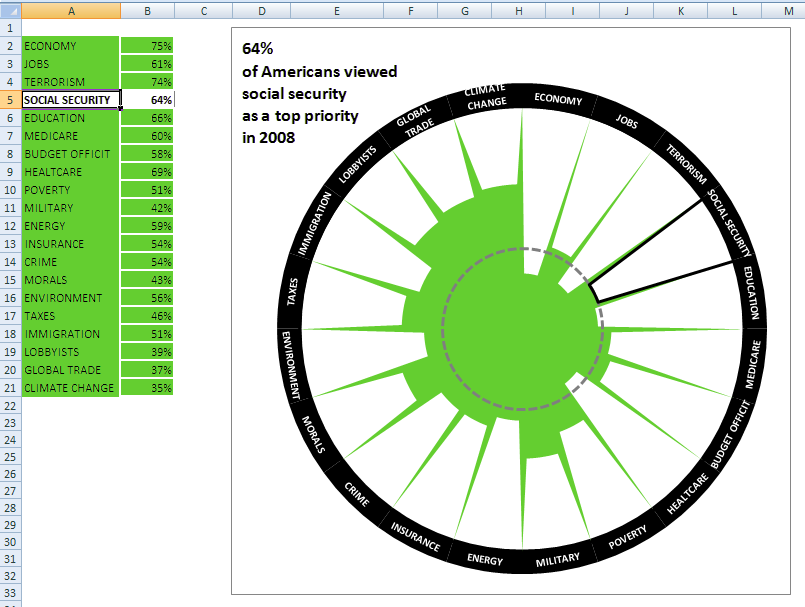picture 1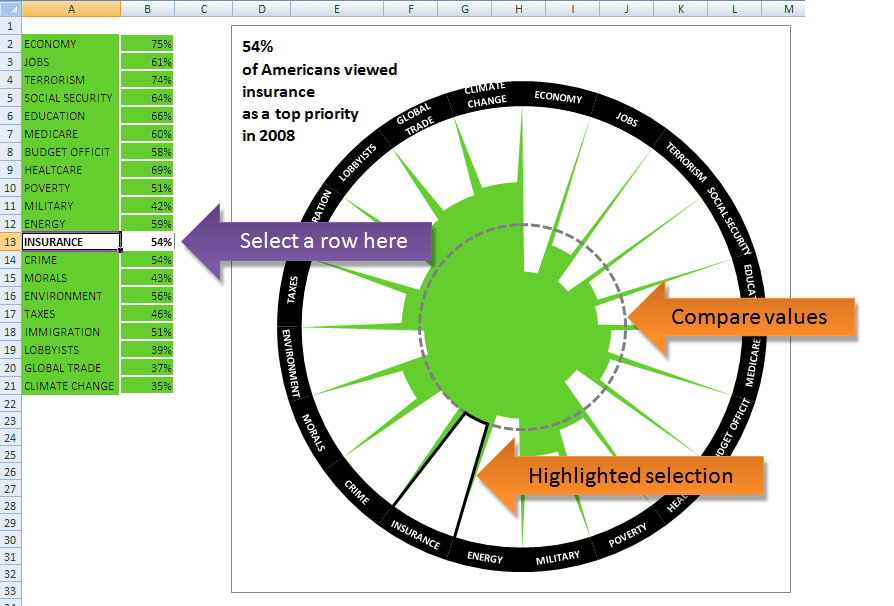picture 2

Can Excel be a creative tool to create advanced, infographic-styled charts? Our answer is YES!

We created some interesting charts combining different chart types and using the built-in features in a bit of an unusual way.

In this article we would like to show how to create the chart you can see above, and how you  can make it dynamic, based on the cell selected by the user.

The data we use on the chart comes from this infographic:
http://awesome.good.is/transparency/web/1010/political-climate-chart/interactive.html

The numbers are percentages representing the fraction of the people with regard to the particular issue that is the top priority.

We designed a chart a similar to the original one, because the circle format could visualize this kind of data  more effectively than a linear one. All of the 20 measured  issues (categories) are visible on the chart now, but you can add more data to the table. The names in the file are built to be dynamically growing, so the new data will immediately appear on the chart.

To make the comparison easier
we turned the chart inside out and used active-cell based highlighting together with a marker line.

Chart components

On the chart you will find 4 data series, three of which are filled with the radar type chart, and the fourth one is a doughnut chart with a  label. The dynamic chart title serves to make the chart more “readable”.

It is important to note that nothing is drawn manually.  The only pain is that Excel cannot set the angle of the label added with the help of the doughnut series, so it is adjusted manually.

• The white columns growing from outside to the centre of the circle represent the data. But the series itself is the green area – it “cuts out” the data columns from the white background of the radar chart.
• The black outline around a white column highlights the data selected by the active cell (row)
• The grey dotted circle is a contour-line and it indicates the value of the category to make comparable with the others.

Doughnut data series:

• The black circle at the outer rim was created as doughnut-type which makes creating the labels easier.

Primary data display – inside-out columns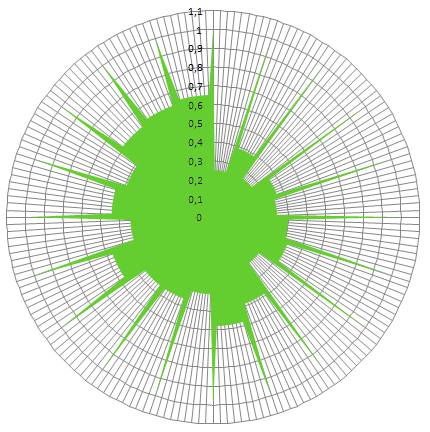picture 3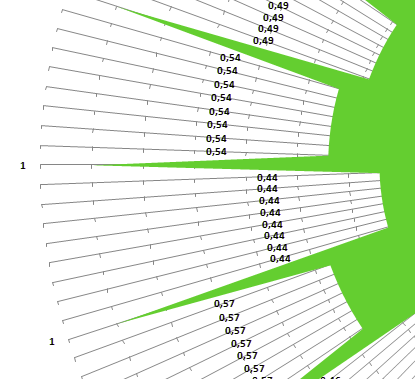picture 4

The whole chart is inside-out, so we need the data for series as 1 – <percentage value>. The gridlines may help to find out what is happening – you can see by counting on picture 4 that there are 8 data points for each category value (data column) plus one for the gap between the columns. So the total number of data points = 9 * number of categories.

The array for the green data series should look something like this: (remember, the data here is 1 – <percentage value>)

1

0.25

0.25

0.25

0.25

0.25

0.25

0.25

0.25

1

0.39

0.39

0.39

0.39

0.39

0.39

0.39

0.39

1

0.26

0.26

.

.

.

How can this array been created as a named formula – without using helper cells on the worksheet?

Capturing input data

First we define the parameters of the data series:

 Named formula Note Refers to n_ColPoints Predefined number of data points for one category + 1 point for the gap =9 n_Category Number of categories in the data table. In the example n_Category = 20 =COUNTA( Sheet1!\$A\$1:\$A\$100 ) n_TotPoints Total number of data points on the radar chartIn the example n_TotPoints = 180 =n_ColPoints * n_Category

You can change the value of n_ColPoints - lowering it will result in a more triangular-shaped column, increasing will diminish the gap. In the example we use 9, as this gives a good result regarding visibility.

Note: Number of categories are limited to 100 in the dynamic named formulae, however the number of effectively displayable categories is far less.

Create a dynamic range for the input data:

 rng_h n_Category could be used in the INDEX formula to make the data table dynamic =Sheet1!\$B\$2:INDEX( Sheet1!\$B\$2:\$B\$100, n_Category)

Building the main data series

And here comes a bit more difficult part…

The structure of the array we would like to have is simple: a gap data followed by the 1st category data repeated 8 times, gap again, 2nd category data repeated 8 times, gap, etc.

How can we imagine it?

Step 1 – Repeated values for the chart columns

First of all, let’s see that if we can separate the problem of category data from the problem of gap points! If we build up an array without gaps but having 9 times the category data, it will not be a big deal to change the 1st, 10th, 19th, 18th, 37th,…,172nd data to the gap-value.
So let’s start with this array as intermediate result – every value listed 9 times, and there is 20 category values, so we need an array of 180 elements:

0.75

0.75

0.75

0.75

0.75

0.75

0.75

0.75

0.75

0.61

0.61

0.61

0.61

0.61

0.61

0.61

0.61

0.61

0.74

0.74

0.74

.

.

.

And what do we have as input?

The name rng_h contains the input data as a vector of 20 category values:

0,75

0,61

0,74

.

.

.

So we want to have a one-dimensional vector of 180 elements using another one-dimensional vector with 20 elements and we need to repeat each element nine times. A very powerful function of Excel could be used in this case: MMULT ! What is the array we should multiply by our rng_h to have the above mentioned result? If you are familiar with the rules of matrix multiplication, you will easily find the answer: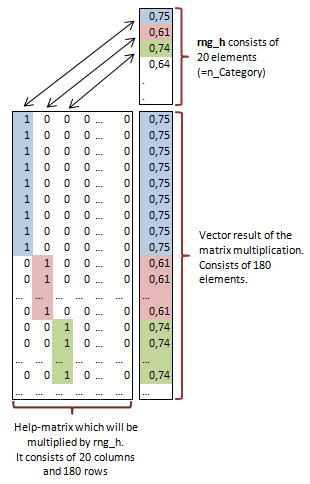Step 2 – Build a helper matrix for the value selection

The next step is to find a way to build up this 180 x 20 sized matrix of 1/0 values. It is simple if you see the pattern: 1 = TRUE, 0 = FALSE, so this matrix is a 2D representation of which data point belongs to which category in the 180 length array: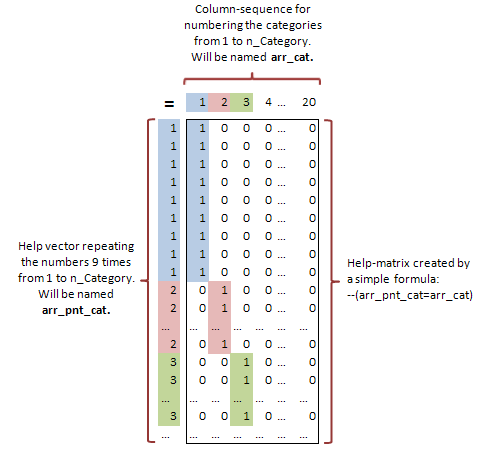Step 3 – Build a helper array for the value addressing

Now we are close! We need the left-hand-side vector from the above picture which tells us which category the data point belongs to. It consists of 180 elements: repeating number 1 nine times, number 2 nine times, going up to 20 repeated nine times. We can create it easily using the INT formula!

Let’s summarize what we have now!

We will need two helping-vectors:

 arr_pnt row-sequence to number the data points from 1 to n_TotPoints {1;2;3;….;180} =ROW( Sheet1!\$A\$1: INDEX(Sheet1!\$A: \$A,n_TotPoints)) arr_cat column-sequence to number the categories from 1 to n_Category {1,2.3,…..,20} =COLUMN( OFFSET(Sheet1!\$A\$1,,,,n_Category))

Determine the structure of the data points:

 arr_pnt_cat this array – as a sequence – identifies which category the data point belongs to. It consists of 180 numbers, repeating 9 times each number from 1 to n_Category: {1;1,1;1;1;1;1;1;1;2;2;2……..;20;20} =INT( (arr_pnt-1) / n_ColPoints )+1or an other solution:=MATCH( arr_pnt, arr_gap, 1 )(see arr_gap below)

So here is the formula for the 1/0 matrix from the first picture, with double negation to convert the TRUE-FALSE to 1/0: –(arr_pnt_cat=arr_cat)

And the matrix multiplication will create us the array without gaps:
MMULT(–(arr_pnt_cat=arr_cat), rng_h)

Step 4 – Inserting gap values

To create the gap values, we need to change the 1st, 2nd…172nd value of this array to 0. The idea is this: if the sequence number of the data point (arr_pnt) is an element of the set of gaps then it should be 0. So we will need the set of gaps:

 arr_gap sequence number of data points used to separate the categories (the gap) {1;10,19;28;37;…..;172} =(ROW(rng_h)- MIN(ROW(rng_h)))*n_ColPoints+1

With a MATCH and ISERROR we can have the 0s for the gap points:
ISERROR(MATCH(arr_pnt, arr_gap, 0)

One minus this whole array turns the series inside outSo here is the final named formula for the main data series!

 arr_01 Data series representing the inside-out columns. Repeats 1 minus data percentage 8 times alternating gap points with value 1. =1-MMULT(–(arr_pnt_cat=arr_cat),rng_h)*ISERROR(MATCH(arr_pnt,arr_gap,0))

After creating a filled radar chart adding arr_01 to data series you should see something like on picture 3. Please change the maximum of the axis to 1,1.  The minimum should be 0.

Category labels

Now we have a static inside-out chart. The easiest way to add data labels is by using a doughnut chart with number of slices equal to the number of categories. We will need a very simple array as data series for this doughnut consists of 1s for each category. Power of 0 would not be a solution in this case, because Excel interprets 0^0 as #NUM error, so if we have 0 value or missing data in rng_h, the doughnut ring chart will not be in line with the columns – that is the reason why we use ISNUMBER here, and *1 converts the boolean values to numbers.

 label_1 ISNUMBER for the data range rng_h gives back TRUE where we have data. You need to multiply by 1 to convert it to numeric values: {1;1,1;…;1} =ISNUMBER(rng_h)*1

Add label_1 to the chart series, change the chart type to Doughnut and set the hole size to maximum.

Create this named range for labels:

 labels Dynamic range for labels =OFFSET(rng_h,,-1)

And insert it to the series formula of the ring via the formula bar:
=SERIES( “label_1″,

Now you can add labels to the chart, you need to change it to show the category names. Unfortunately Excel can not adjust the angle of the labels according to the slices – the labels must be rotated manually.

Active-cell based highlight

Excel’s CELL formula without the second parameter makes it possible to determine the active cell. (You can read more about it here.)

 active_row The formula gives back the row number within the data table. =CELL(“row”)- CELL(“row”,rng_h)+1

Now we build up the black outline highlighting the data of the active category. We do not take care of the rest of the categories, so all these data must be zero. We use only the part of the data point-category array (arr_pnt_cat) where the numbers are equal to the number of the active category:

 arr_act_cat This array contains 0s for all the categories except the active row-category (9 times 1). =(arr_pnt_cat=active_row) * arr_pnt_cat

With exactly the same logic used to build up the main data series we can create the data series for the black outline:

 arr_a1 Comparing to arr_01 the only difference is in the 1-0 matrix: here we use the active-category based arr_ac t_cat instead of arr_pnt_cat which contains all of the indexes. =1-MMULT(–(arr_act_cat=arr_cat),rng_h)* ISERROR(MATCH(arr_pnt,arr_gap,0))

Add the arr_a1 series to the chart. It should be a filled radar chart. Set the marker fill colour to none but set a line at least 2,5 pt wide with black colour.

Active-cell based circle

This circle represents the value of the active category and makes the comparison easier . The only thing to do is to set up a series that contains this value for all of the 180 data points.
 arr_a2 Choose the active data from the data table using INDEX and create a vector of this data by multiplying with a 1-vector. If the active row is not in the data table, we do not need this circle, this is why multiplied with is_in_rng =(1 – arr_pnt ^ 0* INDEX(rng_h,active_row))* is_in_rng

Where is_in_rng is:

 is_in_rng We have chosen this nice formula to determine if the active row is within the data range or not. If the active row is below the table, MEDIAN gives back the last row of the table, so the equation will be false. Similarly when the active row is above the table MEDIAN gives the first row of rng_h (which is CELL(“row”,rng_h) so the equation will be false too. =CELL(“row”)= MEDIAN( CELL(“row”), CELL(“row”,rng_h), MAX(ROW(rng_h)))

Add the series arr_a2 to the chart. It should be filled radar. Set the marker fill colour to none but set a line at least 2,5 pt wide with black colour and dotted style.

Because cell selection does not trigger calculation, you need to add a one-liner code to the worksheet object. In the VB Editor click on the sheet name under your project, and copy this code to the code window:

Private Sub Worksheet_SelectionChange(ByVal Target As Range)

Target.Parent.Calculate

End Sub

It tells Excel to calculate the worksheet for each and every selection change.

Dynamic Title

Only one thing left: to make the chart title dynamic showing the name and value from the active row.

 TT This name will be added to the chart title. The formula itself is a concatenation on the active data, the name of active category and some line breaks CHAR(10). =IF(is_in_rng,TEXT(OFFSET(OFFSET(Sheet1!\$A\$1,active_row,),,1),”0%”)& CHAR(10) & “of Democrats viewed” & CHAR(10) & LOWER(OFFSET(Sheet1!\$A\$1,active_row,)) & CHAR(10) & “as a top priority” & CHAR(10)&”in 2011″,””)

On the formula bar assign the name TT to the chart title box.

And you can find some more charts with this technique here: